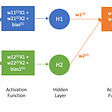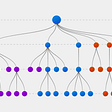# [ML Crash Course] (Stochastic) Gradient Descent

Suppose we had enough time and computing resources to calculate the loss for all possible values of w1. For the kind of regression problems we’ve been examining, the resulting plot of loss vs. w1 will always be convex. In other words, the plot will always be bowl-shaped, kind of like this:

Convex problems have only one minimum; that is, only one place where the slope is exactly 0. That minimum is where the loss function converges.

Calculating the loss function for every conceivable value of w1 over the entire data set would be an inefficient way of finding the convergence point. Let’s examine a better mechanism — very popular in machine learning — called gradient descent.

The first stage in gradient descent is to pick a starting value (a starting point) for w1. The starting point doesn’t matter much; therefore, many algorithms simply set w1 to 0 or pick a random value. The following figure shows that we’ve picked a starting point slightly greater than 0:

The gradient descent algorithm then calculates the gradient of the loss curve at the starting point. The gradient of the loss is equal to the derivative (slope) of the curve, and tells you which way is “up” or “down”. When there are multiple weights, the gradient is a vector of partial derivatives with respect to the weights.

Don’t worry too much even if you don’t know how to calculate partial derivatives. At this stage, you just need to understand what’s going on here.

Note that a gradient is a vector, so it has both of the following characteristics:

• a direction
• a magnitude

The gradient always points in the direction of the steepest increase in the loss function. The gradient descent algorithm takes a step in the direction of the negative gradient in order to reduce loss as quickly as possible. To determine the next point along the loss function curve, the gradient descent algorithm adds some fraction of the gradient’s magnitude to the starting point as shown in the following figure:

The gradient descent then repeats this process, edging ever closer to the minimum.

# Reducing Loss: Learning Rate

As noted, the gradient vector has both a direction and a magnitude. Gradient descent algorithms multiply the gradient by a scalar known as the learning rate (also sometimes called step size) to determine the next point. For example, if the gradient magnitude is 2.5 and the learning rate is 0.01, then the gradient descent algorithm will pick the next point 0.025 away from the previous point.

Hyperparameters are the knobs that programmers tweak in machine learning algorithms. Most machine learning programmers spend a fair amount of time tuning the learning rate. If you pick a learning rate that is too small, learning will take too long:

Conversely, if you specify a learning rate that is too large, the next point will perpetually bounce haphazardly across the bottom of the well like a quantum mechanics experiment gone horribly wrong:

There’s a just-right learning rate for every regression problem. This value is related to how flat the loss function is. If you know the gradient of the loss function is small then you can safely try a larger learning rate, which compensates for the small gradient and results in larger step size.

# Reducing Loss: Stochastic Gradient Descent

In gradient descent, a batch is the total number of examples you use to calculate the gradient in a single iteration. So far, we’ve assumed that the batch has been the entire data set. When working at Google scale, data sets often contain billions or even hundreds of billions of examples. Furthermore, Google data sets often contain huge numbers of features. Consequently, a batch can be enormous. A very large batch may cause even a single iteration to take a very long time to compute.

A large data set with randomly sampled examples probably contains redundant data. In fact, redundancy becomes more likely as the batch size grows. Some redundancy can be useful to smooth out noisy gradients, but enormous batches tend not to carry much more predictive value than large batches.

What if we could get the right gradient on average for much less computation? By choosing examples at random from our data set, we could estimate (albeit, noisily) a big average from a much smaller one. Stochastic gradient descent (SGD) takes this idea to the extreme — it uses only a single example (a batch size of 1) per iteration. Given enough iterations, SGD works but is very noisy. The term “stochastic” indicates that the one example comprising each batch is chosen at random.

Mini-batch stochastic gradient descent (mini-batch SGD) is a compromise between full-batch iteration and SGD. A mini-batch is typically between 10 and 1,000 examples, chosen at random. Mini-batch SGD reduces the amount of noise in SGD but is still more efficient than full-batch.

To simplify the explanation, we focused on gradient descent for a single feature. Rest assured that gradient descent also works on feature sets that contain multiple features.

--

--

--

## More from A Ydobon

Ydobon is nobody.

Love podcasts or audiobooks? Learn on the go with our new app.

## TensorFlow Extended 101: (literally) Everything you need to know## Here’s How I Predicted Apple’s Stock Price Using Natural Language Processing## Introduction to Generative Adversarial Networks(GANs)## A quick guide to understanding Support Vector Machines (SVM)## NLP Coaching — The Difference That Makes a Bond## ML Model — Linear Regression## What is Apple Neural Engine and why it’s important## A Ydobon

Ydobon is nobody.

## What exactly is a back propagation technique?